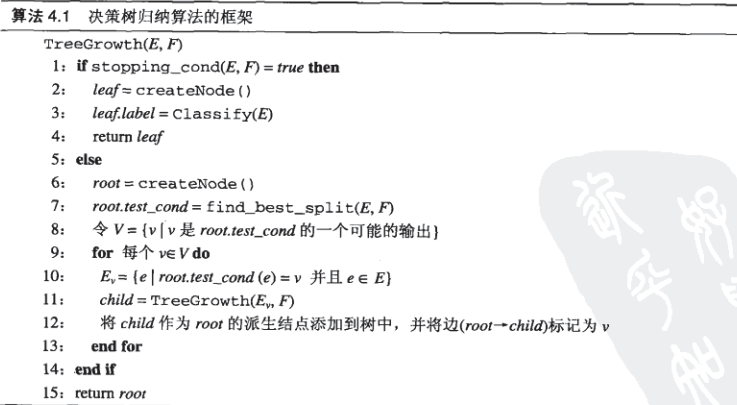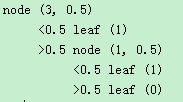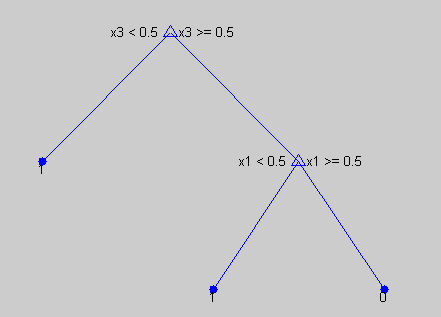• maketree函数递归建立 tree=struct(‘isnode’,1,‘a’,0.0,‘mark’,0.0,‘child’,{}) 所有分支递归的存在child{}中 函数gan（D）计算D的信息率 代码可以自动适应不同的分类标签和类别个数 代码 function tree=...
代码解释
maketree函数递归建立树 tree=struct(‘isnode’,1,‘a’,0.0,‘mark’,0.0,‘child’,{}) 所有分支递归的存在child{}中 函数gan（D）计算D的信息率 代码可以自动适应不同的分类标签和类别个数
代码
function tree=maketree(D,a)
tree=struct('isnode',1,'a',0.0,'mark',0.0,'child',{});%isnode判断是否是分支还是叶子，a表示节点属性，若节点是叶子，a表示分类结果，child是孩子
tree(1).a=1;%给tree分配一个确切的内存
if length(unique(D(:,end)))==1%D中样本属于同一类别
tree.isnode=0;%把tree标记为树叶
tree.a=D(1,end);%把tree的类别标记为D的类别
return
end

if sum(a)==0 ||length(D)==0 %属性划分完毕
tree.isnode=0;%把tree标记为树叶
tree.a=mode(D(:,end));%把tree的类别标记为D出现最多的类别
return
end
for i=1:length(a)
if a(i)==1
if length(unique(D(:,i)))==1
tree.isnode=0;%把tree标记为树叶
tree.a=mode(D(:,end));%把tree的类别标记为D出现最多的类别
return
end
end
end
gain=zeros(length(a),1); %保存每个属性的信息增益
best=zeros(length(a),1); %保存每个属性的最佳划分

for i=1:length(a)
if a(i)==1
t=D(:,i);
t=sort(t);

gain1=zeros(length(t)-1,1);
for j=1:length(t)-1%二分划分
ta=(t(j)+t(j+1))/2;

Df=D(D(:,i)<=ta,:);
Dz=D(D(:,i)>ta,:);
gain1(j)=ent(D)-(ent(Df)*length(Df(:,end))/length(D(:,end))+ent(Dz)*length(Dz(:,end))/length(D(:,end)));
end

[gain(i),j]=max(gain1);
ta=(t(j)+t(j+1))/2;
best(i)=ta;
end
end
[g,m]=max(gain);%选择信息增益最大的属性
D1=D(D(:,m)<=best(m),:);
D2=D(D(:,m)>best(m),:);
a(m)=0;

tree.a=best(m); %建立分支
tree.mark=m;
% disp('****************************')
% tree.a
% tree.mark
tree.isnode=1;
tree.child(1)=maketree(D1,a);
tree.child(2)=maketree(D2,a);

end
function f=ent(D)%计算信息商
l=unique(D(:,end));
if length(D)==0
f=0;
return
end
f=0;
t=zeros(length(l),1);
for i=1:length(D(:,end))
for j=1:length(l)
if D(i,end)==l(j)
t(j)=t(j)+1;
break;
end
end
end
n=length(D(:,end));
for i=1:length(l)
f=f+(t(i)/n)*log2(t(i)/n);
end
f=-f;
end

测试结果
在训练集测试结果精度是0.91 该测试函数是测试随机森林的函数，但不影响测试决策树。
function randomforest()
clc
clear all
T=1;%bagging采样的次数
M = importdata('D:\毕业设计\数据集1\australian.txt');  %读取数据
[sm,sn]=size(M);
% for i=1:sm             %归一化
%     mins=min(M(i,1:sn-1));
%     maxs=max(M(i,1:sn-1));
%     for j=1:sn-1
%         M(i,j)=2*(M(i,j)-mins)/(maxs-mins)-1;
%     end
% end
indices=crossvalind('Kfold',M(1:sm,sn),10); %十折交叉，划分训练集和测试集
testindices=(indices==1); %测试集索引
trainindices=~testindices;%训练集索引
trainset=M(trainindices,:); %获取训练集
testset=M(testindices,:);%获取测试集
[testm,~]=size(testset);
[trainm,trainn]=size(trainset);

predict=zeros(trainm,T);
for t=1:T %开始bagging采样
D=[];%训练集
for i=1:trainm%采样
k=randperm(trainm,1);
D=[D;trainset(k,:)];
end
[~,sn]=size(D);
a=ones(sn-1,1);%属性集合a，1代表该属性未被划分

tree=maketree(D,a);%递归构造简单决策树

for i=1:trainm
treet=tree;
while 1

if treet.isnode==0
predict(i,T)=treet.a;
break;
end
if trainset(i,treet.mark)<=treet.a
treet=treet.child(1);
else
treet=treet.child(2);
end

end
end

end
acc=0;
for i=1:trainm
if trainset(i,end)==mode(predict(i,:))
acc=acc+1;
end
end
acc=acc/trainm

end


展开全文分类
• 决策树算法matlab实现，构造分类决策树并用决策树对模式进行分类识别
• 机器学习决策树MATLAB实现，帮助深刻理解决策树
• matlab实现ID3决策树代码，例程中使用西瓜数据集，运行decisionTree.m即可
• ## 决策树算法 MATLAB 简单实现

万次阅读 多人点赞 2018-10-24 19:22:51
决策树算法 前言 最近在数据挖掘与机器学习的课程上刚刚学到了决策树...文章目录决策树算法前言1 算法流程2 程序设计3 MATLAB 中的调用4 Python 中的调用 1 算法流程 通过阅读机器学习的书籍首先了解决策树算法的基...
决策树算法
前言
最近在数据挖掘与机器学习的课程上刚刚学到了决策树算法，于是，想自己用 MATLAB 简单实现一下。虽然拿其中最简单算法的进行实现，但是，从构思–编写–初步完成，也花费了不少时间，毕竟只有动手编写，才能真正体会到算法的内涵。

文章目录
决策树算法前言1 算法流程2 程序设计3 MATLAB 中的调用4 Python 中的调用

1 算法流程
通过阅读机器学习的书籍首先了解决策树算法的基本思想：通过递归的方式构建一棵树，子树是通过选取某一属性，按照其属性值进行划分产生的。其算法伪代码如下：2 程序设计
程序设计必须对算法的每个细节都要搞清楚，有时可能要实现一个健全完善的算法很困难，我们可以对算法进行简化，忽略复杂的情况，比如，在上面的构建决策树算法的步骤中，子树的划分可能有多个输出，连续属性和无序离散属性的划分的方法也有所不同，如果都要将这些考虑进去程序的设计难度会很大。作为初学者，可以对问题进行简化：
假设无序离散属性都只是二元属性，属性值用0或1表示类别只有两类，用0或1表示每个节点只有两个输出
在明确了细节之后，还需考虑另外一个问题：数据结构。在程序中用什么数据结构来描述所构建的“树”？这一步很关键，因为在对训练集之外的记录进行测试的时候要用到该数据结构。
由于自己实现决策树算法的目的只是加深对算法的理解，并不是实际开发，因此，只是将“树”的结构和参数打印出来。
function build_tree(x, y, L, level, parent_y, sig, p_value)
% 自编的用于构建决策树的简单程序，适用于属性为二元属性，二分类情况。（也可对程序进行修改以适用连续属性）。
% 输入：
% x：数值矩阵，样本属性记录（每一行为一个样本）
% y：数值向量，样本对应的labels
% 其它参数调用时可以忽略，在递归时起作用。
% 输出：打印决策树。
if nargin == 2
level = 0;
parent_y = -1;
L = 1:size(x, 2);
sig = -1;
p_value = [];
%        bin_f = zeros(size(x, 2), 1);
%        for k=1:size(x, 2)
%            if length(unique(x(:,k))) == 2
%               bin_f(k) = 1;
%            end
%        end
end
class = [0, 1];
[r, label] = is_leaf(x, y, parent_y); % 判断是否是叶子节点
if r
if sig ==-1
disp([repmat('     ', 1, level), 'leaf (', num2str(label), ')']);
elseif sig ==0
disp([repmat('     ', 1, level), '<', num2str(p_value),' leaf (', num2str(label), ')']);
else
disp([repmat('     ', 1, level), '>', num2str(p_value),' leaf (', num2str(label), ')']);
end
else
[ind, value, i_] = find_best_test(x, y, L); % 找出最佳的测试值
%
%         if ind ==1
%            keyboard;
%         end

[x1, y1, x2, y2] = split_(x, y, i_, value); % 实施划分
if sig ==-1
disp([repmat('     ', 1, level), 'node (', num2str(ind), ', ', num2str(value), ')']);
elseif sig ==0
disp([repmat('     ', 1, level), '<', num2str(p_value),' node (', num2str(ind), ', ', num2str(value), ')']);
else
disp([repmat('     ', 1, level), '>', num2str(p_value),' node (', num2str(ind), ', ', num2str(value), ')']);
end
%         if bin_f(i_) == 1
x1(:,i_) = [];
x2(:,i_) = [];
L(:,i_) = [];
%             bin_f(i_) = [];
%         end
build_tree(x1, y1, L, level+1, y, 0, value); % 地柜调用
build_tree(x2, y2, L, level+1, y, 1, value);
end

function [ind, value, i_] = find_best_test(xx, yy, LL) % 子函数：找出最佳测试值（可以对连续属性适用）
imp_min = inf;
i_ = 1;
ind = LL(i_);
for i=1:size(xx,2);
if length(unique(xx(:,i))) ==1
continue;
end
%            [xx_sorted, ii] = sortrows(xx, i);
%            yy_sorted = yy(ii, :);
vv = unique(xx(:,i));
imp_min_i = inf;
best_point = mean([vv(1), vv(2)]);
value = best_point;
for j = 1:length(vv)-1
point = mean([vv(j), vv(j+1)]);
[xx1, yy1, xx2, yy2] = split_(xx, yy, i, point);
imp = calc_imp(yy1, yy2);
if imp<imp_min_i
best_point = point;
imp_min_i = imp;
end
end
if imp_min_i < imp_min
value = best_point;
imp_min = imp_min_i;
i_ = i;
ind = LL(i_);
end
end
end

function imp = calc_imp(y1, y2) % 子函数：计算熵
p11 = sum(y1==class(1))/length(y1);
p12 = sum(y1==class(2))/length(y1);
p21 = sum(y2==class(1))/length(y2);
p22 = sum(y2==class(2))/length(y2);
if p11==0
t11 = 0;
else
t11 = p11*log2(p11);
end
if p12==0
t12 = 0;
else
t12 = p12*log2(p12);
end
if p21==0
t21 = 0;
else
t21 = p21*log2(p21);
end
if p22==0
t22 = 0;
else
t22 = p22*log2(p22);
end

imp = -t11-t12-t21-t22;
end

function [x1, y1, x2, y2] = split_(x, y, i, point) % 子函数：实施划分
index = (x(:,i)<point);
x1 = x(index,:);
y1 = y(index,:);
x2 = x(~index,:);
y2 = y(~index,:);
end

function [r, label] = is_leaf(xx, yy, parent_yy) % 子函数：判断是否是叶子节点
if isempty(xx)
r = true;
label = mode(parent_yy);
elseif length(unique(yy)) == 1
r = true;
label = unique(yy);
else
t = xx - repmat(xx(1,:),size(xx, 1), 1);
if all(all(t ==0))
r = true;
label = mode(yy);
else
r = false;
label = [];
end
end
end
end

利用MATLAB提供的数据集进行测试，并与 MATLAB 自身提供的决策树分类的函数进行对比。
clc
clear all
load ionosphere % contains X and Y variables
x = X(:,1:3);
ind = x(:,3)>0;
x(ind,3) = 1;
x(~ind,3) = 0;

y = zeros(size(Y));
y(ismember(Y, 'b')) = 1;

ctree = fitctree(x, y);
view(ctree,'mode','graph') % graphic description
% [label, score] = predict(ctree, X(5,:))

build_tree(x, y);

自编程序运行结果
含义说明：
node(属性序号， 划分点)
leaf(类别)MATLAB提供的函数的运行结果结果与MATLAB中自己实现的函数运行结果相同。
3 MATLAB 中的调用
自己对算法的实现的目的主要还是用于加深对算法的理解，但是在实际应用时，还得借助成熟的机器学习工具包，比如MATLAB或Python提供的机器学习工具包。下面介绍一下MATLAB中决策树算法的相关函数的调用方法。
tree = fitctree(x,y)
tree = fitctree(x,y,Name,Value)

根据给定的记录的属性x，对应类别y，构造决策树（二叉树）。要求x为数值矩阵，y为数值向量或cell数组。name-value pair 为可选参数，用于指定算法的参数（划分准则，叶子节点最少记录值等）。x, y 每一行为一个样本。
返回tree为决策树的数据结构。
利用tree进行分类：
label = predict(tree, x)

4 Python 中的调用
scikit-learn 库提供了决策树分类和回归的方法.
训练
>>> from sklearn import tree
>>> X = [[0, 0], [1, 1]]
>>> Y = [0, 1]
>>> clf = tree.DecisionTreeClassifier()
>>> clf = clf.fit(X, Y)

分类
>>> clf.predict([[2., 2.]])
array()

DecisionTreeClassifier能够进行二元分类(标签为[- 1,1])和多类分类(标签为[0，…，K-1])。
展开全文Python
• 1.亲测MATLAB2014a及2016a可运行 2.拥有从训练到测试分类性能的完整流程
• 决策树matlab实例代码赫林格树Matlab 注意：这仍然是高度实验性的，可能容易出错，请谨慎使用！ 基于Cieslak等人的论文“ Hellinger距离决策树是鲁棒且不倾斜的”，该Matlab实现了具有不平衡数据和数值属性的二元...

决策树的matlab实现(运用在手写数字的0，1识别)
单个决策树的实现。
一、载入实验数据
%载入实验的原始数据
images_0=images(:,labels==0);
images_1=images(:,labels==1);
Images_te_0=Images_te(:,Labels_te==0);
Images_te_1=Images_te(:,Labels_te==1);
%对原始数据进行处理
X=[images_0 images_1]'; %将0和1的训练样本拼接成新的矩阵。注意此时时是每一行代表一个样本。
Y=[zeros(5923,1) ones(6724,1)]; %生成训练样本相应的类别标签，是一个列向量。每一个元素代表相应样本所属于的类别。
Images_te_0=Images_te_0'; %测试样本的数字0，每一行代表一个样本。
Images_te_1=Images_te_1'; % 测试样本的数字1，每一行代表一个样本.
二、构建决策树
t=treefit(X,Y);
% X是训练样本，一定注意是每一行代表一个样本数据。Y是训练样本
% 所对应的类别标签，仍旧是一个列向量。t就是根据训练样本构建的一个决策树。
三、用决策树对测试样本进行分类
result0=treeval(t,Images_te_0');
% 对测试样本手写数字0进行识别。result0为一个列向量，每一元素表示对应样本被识别为的类。
%treeval函数的格式为treeval(t,X)，其中，t为构建的决策树，X为测试样本。一定要注意X的每一行表示的是一个样本。
result1=treeval(t,Images_te_1'); %对测试样本数字1进行分类。
四、错误率分析
error0=find(result~=0);
error1=find(result~=1); %找出被识别错误的数字0和1的索引。
rate0=size(error0,1)/980;
rate1=size(error1,1)/980; %分别计算错误率。
实验结果为

展开全文• 程序主要实现对数据的加载和处理，首先加载数据，本算法选择的数据集是鸢尾花数据集，加载的数据形式是元胞数组，本程序先把其转换成字符串数组，后对字符串数组进行处理，将数据部分和标签部分分隔开，数据部分...
• [机器学习]决策树算法的MATLAB实现 这是一篇关于决策树算法的MATLAB实现的文章，也是我的课堂实验，学习的书籍为西瓜书。此文章包含树的建立(使用信息增益，基尼指数)，绘图，预测以及剪枝（后剪枝），部分代码为...
[机器学习]决策树算法的MATLAB实现 这是一篇关于决策树算法的MATLAB实现的文章，也是我的课堂实验，学习的书籍为西瓜书。此文章包含树的建立(使用信息增益，基尼指数)，绘图，预测以及剪枝（后剪枝），部分代码为老师提供。文章中所有的代码以及老师提供的代码以及实验的要求都在以下连接，需要可以自取。应该说，最好就是跟着实验要求去做，然后不懂或者看不明白再来看这里面的代码，应该会对决策树有更加深刻的了解。假如大家对决策树有什么不了解，可以问我，我会尽量解答（当然我属实能力有限，毕竟才开始学，一起努力吧，冲冲冲）当然代码略微杂乱。而且matlab现在似乎提供了决策树等算法的生成包，大家可以自行查略，本文并不涉及。以下为正文
链接：https://pan.baidu.com/s/1ioCVZTNyCZiD0GM9Vy3L4w
提取码：v432
当然我的csdn资源中应该也有这个资源（写完这个应该就会去上传吧）
大家如果觉得还不错可以支持一下

一 .内容
基于 西瓜数据集2.0 的ID3决策树算法实现 数据集watermelon.mat,来自教材中的西瓜数据集2.0，共有18个样本数据。实验中，选取其中的16个样本构成训练集，其余2个样本构成测试集。基于ID3算法采用训练样本构造决策树，并简单绘出生成的决策树。最后，测试该决策树对测试样本的类别划分情况。  基于 Breast Cancer癌症数据集 分析ID3决策树的分类精度 数据集breastcancer.mat中，有277个样本数据，每个数据有9个属性特征以及一个类别标签。基于前述构造ID3决策树的算法程序，试采用10次10折交叉验证法评估ID3决策树模型在此数据集上的分类精度（注：分类精度的度量方法参见教材P29公式2.5）。 (这一部分我的最后结果是90的正确率还是挺开心的嘿嘿。 (不剪枝的话应该66左右。剪枝nb！
二.具体实现 ##基于西瓜数据集2.0的ID3决策树算法实现 （一）要求. 数据集watermelon.mat,来自教材中的西瓜数据集2.0，共有18个样本数据。实验中，选取其中的16个样本构成训练集，其余2个样本构成测试集。基于ID3算法采用训练样本构造决策树，并简单绘出生成的决策树。最后，测试该决策树对测试样本的类别划分情况。
（二）过程
主函数：导入数据集，划分训练集与测试集，构造决策树 Main_DecisionTree.m子函数ID3()：基于ID3算法构造决策树 ID3.m子函数chooseFeature()：选择信息增益最大的属性特征 chooseFeature.m
function bestFeature=chooseFeature(dataset,~)
% 选择信息增益最大的属性特征
%数据预处理
[N,M]=size(dataset);                %样本数量N
M=M-1;                              %特征个数M
y=strcmp(dataset(:,M+1),dataset(1,M+1)); %标签y(以第一个标签为1)
x=dataset(:,1:M);                   %数据x
gain = (1:M);                       %创建一个数组，用于储存每个特征的信息增益
%bestFeature;                       %最大信息增益的特征
Ent_D=calShannonEnt(y);             %当前信息熵
%计算信息增益
for i=1:M
% 计算第i种属性的增益
temp=tabulate(x(:,i));
value=temp(:,1);            %属性值
count=cell2mat(temp(:,2));  %不同属性值的各自数量
Kind_Num=length(value);     %取值数目
Ent=zeros(Kind_Num,1);
% i属性下 j取值的信息熵
for j=1:Kind_Num
% 在第j种取值下正例的数目
Ent(j)= calShannonEnt( y(strcmp(x(:,i),value(j))) );
end
gain(i)=Ent_D-count'/N*Ent;
end
%随机挑选一个最大值
max_gain=find(gain==max(gain));
choose=randi(length(max_gain));
bestFeature=max_gain(choose);
%%%%============================================
end

（1）对数据进行预处理 这里对数据的标签进行了特殊处理 y=strcmp(dataset(:,M+1),dataset(1,M+1)); %标签y(以第一个标签为1) 因为是二分类问题，所以将标签调整的为logical数组，与第一个相同的为1.不相同的为0，方便后续的计算信息熵的处理。 （2）计算信息熵 先利用tabulate来获取每个属性下的不同取值以及其数量
   temp=tabulate(x(:,i));
value=temp(:,1);            %属性值
count=cell2mat(temp(:,2));  %不同属性值的各自数量

这里以此对每一个属性取值调用calShannonEnt函数来计算信息熵
Ent(j)= calShannonEnt( y(strcmp(x(:,i),value(j))) );

这里y(strcmp(x(:,i),value(j)))是选取了和x(:,i)和value(j)相同的取值对应的y传递给calShannonEnt去计算信息熵 （3）随机选取最大的信息熵
max_gain=find(gain==max(gain));
choose=randi(length(max_gain));
bestFeature=max_gain(choose);

这是为了应对当有多个最大的属性特征时的情况，这里我解决的方法是随机从中随机挑选出一个作为最大值，所以也因此，虽然输入数据相同，但训练输出的树也会因此而可能发生不同。
4.子函数calShannonEnt()：计算信息熵 calShannonEnt.m
function shannonEnt = calShannonEnt(y)
% 计算信息熵
% y对应的标签,为1或0，对应正例与反例
N=length(y);            %标签长度
P_T=sum(y)/N;           %正例概率
P_F=(N-sum(y))/N;         %反例概率
if(P_T==0||P_F==0)
%使得p*log2p为0
shannonEnt = 0;
return
end
shannonEnt=-(P_T*log2(P_T)+P_F*log2(P_F));  %信息熵
end

因为传入的为[1，0]的逻辑数组，所以正例可以直接使用sum/N来计算。后面根据信息熵的约定当p=0信息熵p*log2p为0，所以加入了中间的一句判断，来返回该情况
5.子函数splitDataset()：划分数据集 splitDataset.m 6.子函数print_tree()：遍历决策树 print_tree.m 7.子函数tree_plot()：绘出决策树tree_plot.m 8.利用子函数predict()对测试样本进行分类predict.m文件
对训练样本进行类别划分的代码如下：
%----------------------------------------------
y_test=predict(x_test,mytree,labels);
fprintf('测试样本的分类标签为：');
disp(y_test);
%-----------------------------------------------

（三）实验结果 决策树图像：输出：
测试样本的分类标签为：    '否'    '否'

即对 ‘浅白’ ‘蜷缩’ ‘浊响’ ‘模糊’ ‘平坦’ ‘硬滑’ ‘否’ ‘青绿’ ‘蜷缩’ ‘沉闷’ ‘稍糊’ ‘稍凹’ ‘硬滑’ ‘否’ 这两个样本的预测为都为否，预测结果与原标签相同
##基于Breast Cancer数据集分析ID3决策树的分类精度 （一）要求 数据集breastcancer.mat中，有277个样本数据，每个数据有9个属性特征以及一个类别标签。基于前述构造ID3决策树的算法程序，试采用10次10折交叉验证法评估ID3决策树模型在此数据集上的分类精度（注：分类精度的度量方法参见教材P29公式2.5）。
（二）实验过程 1.predict函数的修改 在predict中，因为数据集的标签不能包含所有标签，所以当测试集中出现了数据集中没有的标签时，将无法进行预判而返回空数组，比如年龄这一个属性在训练集中没有20-39这个属性但出现在测试级中，将会无法返回预测标签。所以对其进行更改为：
hasKeys=0;
keys = node.keys();
for i = 1:length(keys)
key = keys{i};
c = char(feature);
if strcmp(key,c)
queue=[queue,{node(key)}]; %队列变成该节点下面的节点
end
end
key = keys{randi(length(keys))};
queue=[queue,{node(key)}];
end

即随机选取一个属性值进行预判，接下去进行预测
2.主函数 主函数包含3部分：数据读取，10次10折交叉验证，结果输出。因为是2分类，所以数据读取部分中将与第一个标签相同的分为一层，另一个分为另一层。最后将随机采样的得到结果存储在D_index中，其维度为（每个数据组）*10，10个列向量中每一个维度都存这一组原数的索引。在这之中为了数据维度保持一致，放置同一个数组中所以将部分数据舍弃了，保持数据维度一致
% breastcancer数据集
%-----------------数据读取----------------------
clear
clc
size_data = size(breastcancer); %breastcancer 为导入工作台的数据

%-------------10次10折交叉验证-------------------
k_time=10;
crossValidation_time=10;
y_lable=breastcancer(2:size_data(1),size_data(2));
T_P=zeros(k_time,crossValidation_time);
for i=1:crossValidation_time
%分为训练集和测试集(10折)，
y_1=find(strcmp(y_lable(:),y_lable(1)));%与第一个标签相同的为一层次
y_2=find(~strcmp(y_lable(:),y_lable(1)));%其余为另一个层次
y_1_length=length(y_1);
y_2_length=length(y_2);
y_1_perNum=floor(y_1_length/k_time);
y_2_perNum=floor(y_2_length/k_time);
y_1_randIndex=randperm(y_1_length);
y_2_randIndex=randperm(y_2_length);
D_index=zeros(y_1_perNum+y_2_perNum,k_time); %D中存放了10组数据索引
for j=1:k_time                          %有数据被丢弃
D_index(:,j)=[...
y_1(y_1_randIndex(y_1_perNum*(j-1)+1:y_1_perNum*j));...
y_2(y_2_randIndex(y_2_perNum*(j-1)+1:y_2_perNum*j))];
end
D_index=D_index+1;
perNum_D=y_1_perNum+y_2_perNum;
%训练10折交叉验证
for k=1:k_time
%获取此时的数据集以及测试集
x_train = breastcancer(...
[1; reshape(D_index(:,1:k-1),[],1);...
reshape(D_index(:,k+1:k_time),[],1)],:) ;     %这里加上了属性标签行
x_test = breastcancer(D_index(:,k),:);  %选择最后两个当测试集
%训练
size_data = size(x_train);
dataset = x_train(2:size_data(1),:); %纯数据集
labels = x_train(1,1:size_data(2)-1); %属性标签
%生成决策树
mytree = ID3_2(dataset,labels);
%预测测试集标签并计算精度
y_test=predict_2(x_test(:,1:end-1),mytree,labels);
T_P(i,k)=sum(strcmp(y_test',x_test(:,end)))/perNum_D;
end
end
%----------------结果输出-------------------------
fprintf('10次10折交叉验证的精度结果为:\n');
for i=1:10
fprintf('第%d次:%f\n',i,mean(T_P(i,:)));
fprintf('\t%f\t%f\t%f\t%f\t%f\n',T_P(i,1:5));
fprintf('\t%f\t%f\t%f\t%f\t%f\n',T_P(i,6:10));
end
fprintf('平均精度为：%d\n',mean(mean(T_P)));

（三）实验结果
10次10折交叉验证的精度结果为:
第1次:0.618519
0.592593	0.629630	0.518519	0.666667	0.555556
0.444444	0.666667	0.592593	0.777778	0.740741
第2次:0.637037
0.740741	0.666667	0.592593	0.370370	0.592593
0.703704	0.629630	0.666667	0.666667	0.740741
第3次:0.674074
0.703704	0.629630	0.777778	0.703704	0.666667
0.555556	0.703704	0.703704	0.629630	0.666667
第4次:0.655556
0.629630	0.518519	0.629630	0.629630	0.666667
0.703704	0.666667	0.740741	0.666667	0.703704
第5次:0.681481
0.703704	0.777778	0.814815	0.666667	0.740741
0.555556	0.703704	0.814815	0.518519	0.518519
第6次:0.670370
0.629630	0.740741	0.629630	0.740741	0.629630
0.703704	0.703704	0.592593	0.629630	0.703704
第7次:0.677778
0.592593	0.777778	0.666667	0.629630	0.703704
0.740741	0.666667	0.740741	0.555556	0.703704
第8次:0.659259
0.629630	0.629630	0.814815	0.666667	0.851852
0.629630	0.555556	0.666667	0.592593	0.555556
第9次:0.685185
0.703704	0.740741	0.555556	0.703704	0.703704
0.629630	0.666667	0.666667	0.666667	0.814815
第10次:0.670370
0.777778	0.666667	0.740741	0.518519	0.666667
0.740741	0.629630	0.481481	0.740741	0.740741

平均精度为：0.662963 即最后得到的精度为0.662963，多次运行的结果在0.659，0.665的范围左右不会相差太远
（四）改进 1.使用基尼指数来作为选择指标 这里与之前的计算信息增益基本相同，最后选择的指标改为了最小的而非最大
function bestFeature=chooseFeatureGini(dataset,~)
% 选择基尼指数最小的属性特征

%数据预处理
[N,M]=size(dataset);                %样本数量N
M=M-1;                              %特征个数M
y=strcmp(dataset(:,M+1),dataset(1,M+1)); %标签y(以第一个标签为1)
x=dataset(:,1:M);                   %数据x
Gini_index = zeros(1,M);            %创建一个数组，用于储存每个特征的信息增益
%bestFeature;                       %最大基尼系数的特征

%计算基尼指数
for i=1:M
% 计算第i种属性的基尼指数
temp=tabulate(x(:,i));
value=temp(:,1);            %属性值
count=cell2mat(temp(:,2));  %不同属性值的各自数量
Kind_Num=length(value);     %取值数目
Gini=zeros(Kind_Num,1);
% i属性下 j取值的基尼指数
for j=1:Kind_Num
% 在第j种取值下正例的数目
Gini(j)= getGini( y(strcmp(x(:,i),value(j))) );
end
Gini_index(i)=count'/N*Gini;
end
%随机挑选一个最小值
min_GiniIndex=find(Gini_index==min(Gini_index));
choose=randi(length(min_GiniIndex));
bestFeature=min_GiniIndex(choose);
end

用于计算基尼指数的代码：
function Gini = getGini(y)
% 计算基尼系数
% y对应的标签,为1或0，对应正例与反例
%%%%%%========================================
N=length(y);            %标签长度
P_T=sum(y)/N;           %正例概率
P_F=1-P_T;              %正例概率
Gini=1-P_T*P_T-P_F*P_F;  %基尼系数
%%%%%%=====================================
end

在做这部分改动之后，10次10折交叉验证精度影响较小，基本与之前相同。
2.当叶子节点时，找最多的标签值，而非选取第一个 这一步将ID3的部分代码修改如下
size_data = size(dataset);
classList = dataset(:,size_data(2));
%%属性集为空，找最多数
temp=tabulate(classList);
value=temp(:,1);            %属性值
count=cell2mat(temp(:,2));  %不同属性值的各自数量
index=find(max(count)==count);
choose=index(randi(length(index)));
nodeLable =  char(value(choose));
if size_data(2) == 1
myTree =  nodeLable;
return
end

3.在没有对应属性值的情况下，输出当前节点在训练时的最多标签值 这一部分是为了应对训练集中没有测试集的对应属性值，或者后续将该模型用于预测时没有对应属性值的情况。不使用之前的随机选择节点继续，因为这样可能会带来新的误差。比如没有年龄10-19的标签，却随机到了60-69的标签，这样带来更大的误差。而且会出现模型对相同的数据输出不一样标签的情况。所以在leaf中，加入一个标签，用于记录当前节点的数据的最多标签。
leaf('nodeLabel')= nodeLable;

nodeLable为当前节点的数据的最多标签。当然标签不会作为属性值进入预测节点。 所以对预测脚本predict_2.m进行修改：
在 string(class(node))=="containers.Map" %的情况时加入
%除去nodelable标签（不影响检测）
keys = node.keys();
index=find(strcmp(keys,'nodeLabel'));
if(~isempty(index))
keys=[keys(1:(index-1)),keys((index+1):end)];
end

这修改后，10次10折交叉验证精度比之前的65%-66%有小幅度提升，在68%-69%左右
4.去除没有划分能力的节点 当属性只有一种值时，会产生没有划分能力的节点，比如在训练时出现年龄属性中只有10-19的一种属性，那么该属性将剔除而不会被加入训练中。这样可以使树的模型更加简单而且在判断是否为节点时更加容易，边必然有多个keys，而节点只有一个。 对ID3的%全为同一类，熵为0以及%属性集为空这2个部分中间加入
%去除完全相同的属性，避免产生没有分类结果的节点
choose=ones(1,size_data(2));
for i=1:(size_data(2)-1)
featValues = dataset(:,i);
uniqueVals = unique(featValues);
if(length(uniqueVals)<=1)
choose(i)=0;
end
end
labels=labels((choose(1:size_data(2)-1))==1);
dataset=dataset(:,choose==1);

5.后剪枝 这依赖到之前3，加入了leaf(‘nodeLabel’)= nodeLable，即在leaf中加入了原本在训练是当前数据集在这个节点的最多标签值
function [correct,tree_pruning] = pruning(x_V,tree,feature_list)
%-----------------剪枝-------------------------
%correct：返回的数据集的预测值正确程度数组，1为预测正确
%tree_pruning：剪枝后的数组
%x_V：训练集
%tree：剪枝前的树
%feature_list：训练集的标签
if(string(class(tree))~="containers.Map")
%达到叶节点，计算标签与当前数据的真实标签的异同
%将结果保存在correct数组中
correct=strcmp(x_V(:,end),tree)';
tree_pruning=tree;%返回原本的节点
return;
else
size_data = size(x_V);
labels=feature_list;            %数据的属性
Feature=char(tree.keys);        %当前节点的属性
FeatureIndex=strcmp(labels,Feature);%节点属性在所有属性中的索引
FeatureValue=x_V(:,FeatureIndex);   %所有属性
x_V=x_V(:,logical([~FeatureIndex,1])); %删除该特征
feature_list=feature_list(~FeatureIndex);
theTree = containers.Map;%新的节点以及边
theLeaf = containers.Map;
leaf=tree(Feature);%原本的叶子节点
keys=leaf.keys;    %获取属性的取值
%除去nodelable标签（不影响检测）
index=find(strcmp(keys,'nodeLabel'));
if(~isempty(index))
keys=[keys(1:(index-1)),keys((index+1):end)];
end

correct=[];  %数据将包含目前数据预测的正确与否，为0-1数组
for i=1:length(keys)
value=keys{i};
x_V_value=x_V(strcmp(FeatureValue,value),:); %删除拥有特征的数量
if(~isempty(x_V_value))
%数据集里有该取值，计算预测结果正确与否
[correct_per,theLeaf(value)] = pruning(x_V_value,leaf(value),feature_list);
correct=[correct,correct_per];
else
%数据集里没有该取值，保留原本的节点
theLeaf(value)=leaf(value);
end
end
theLeaf('nodeLabel')= char(leaf('nodeLabel'));%获取之前的节点
theTree(Feature) = theLeaf;

acc = sum(correct)/length(correct);%原本的精度
acc_pruning = strcmp(x_V(:,end),leaf('nodeLabel'))/size_data(1);%不划分的精度
if(acc<=acc_pruning)
%假如不划分的精度更高，那么选取原本训练时最多的标签
tree_pruning= char(leaf('nodeLabel'));
else
%保留树
tree_pruning=theTree;
end
end

end

此时需要丢数据进行一些改动： （1）得到验证集用于剪枝 这里选用了，8个数据集用于训练，1个用于验证，1个用于测试
if k~=k_time
x_train = breastcancer(...
[1; reshape(D_index(:,1:k-1),[],1);...
reshape(D_index(:,k+2:k_time),[],1)],:) ;     %这里加上了属性标签行
else
x_train = breastcancer([1; reshape(D_index(:,2:k-1),[],1)],:) ;     %这里加上了属性标签行
end
x_valid = breastcancer(D_index(:,k),:);                 %选择验证集
x_test = breastcancer(D_index(:,mod(k+1,k_time)+1),:);  %选择测试集

（2）用剪枝后的数据集去验证
%剪枝
[correct,tree_pruning] = pruning(x_valid,mytree,labels);
%----------------------------------------------
y_test=predict_2(x_test(:,1:end-1),tree_pruning,labels);
T_P(i,k)=sum(strcmp(y_test',x_test(:,end)))/perNum_D;
%-----------------------------------------------

（五）改进结果 上述所有的改进之后，决策树的精度得到了很大的提升，在89% 90%左右
10次10折交叉验证的精度结果为:
第1次:0.885185
0.925926	0.888889	0.888889	0.888889	0.851852
0.925926	0.925926	0.925926	0.777778	0.851852
第2次:0.914815
0.962963	0.888889	0.962963	0.925926	0.851852
0.851852	0.888889	0.888889	1.000000	0.925926
第3次:0.888889
0.925926	0.888889	0.888889	0.888889	0.851852
0.851852	0.888889	0.851852	0.925926	0.925926
第4次:0.918519
0.888889	0.888889	0.962963	0.962963	0.888889
0.888889	0.851852	0.925926	0.925926	1.000000
第5次:0.896296
0.740741	0.925926	0.962963	0.925926	0.925926
0.851852	0.925926	0.925926	0.888889	0.888889
第6次:0.903704
0.851852	0.888889	0.888889	0.925926	1.000000
0.925926	0.925926	0.814815	0.851852	0.962963
第7次:0.907407
0.962963	0.851852	0.740741	0.925926	0.962963
0.925926	0.925926	0.851852	0.962963	0.962963
第8次:0.918519
0.962963	0.888889	0.888889	0.851852	1.000000
1.000000	0.888889	0.888889	0.962963	0.851852
第9次:0.896296
0.925926	0.925926	0.851852	0.851852	0.925926
0.814815	0.888889	0.962963	0.925926	0.888889
第10次:0.918519
0.925926	0.851852	0.888889	0.925926	0.888889
0.925926	0.962963	0.851852	1.000000	0.962963
平均精度为：0.904815

END 到此结束啦，谢谢你能看到这，感谢。你有自己试着去实现吗？你的精度又是多少呢？886
展开全文机器学习
• 这是一个使用matlab实现决策树cart的算法。
• %% 使用ID3决策树算法预测销量高低 clc; clear ; %% 数据预处理 disp('正在进行数据预处理...'); [matrix,attributes_label,attributes] = id3_preprocess(); %% 构造ID3决策树，其中id3()为自定义函数 disp('数据...机器学习
• 决策树 id3算法实现多叉树树形图显示，使用matlab编程实现多叉树生成结构体，再对结构体进行处理，生成树形图。
• MATLAB实现决策树分类算法 基于python实现决策树 决策树原理概述 决策树通过把样本实例从根节点排列到某个叶子节点来对其进行分类。树上的每个非叶子节点代表对一个属性取值的测试， 其分支就代表测试的每个...python ID3
• 决策树方法对手写数字的识别决策树matlab实现(运用在手写数字的0，1识别)单个决策树实现。一、载入实验数据%载入实验的原始数据images = loadMNISTImages('train-images.idx3-ubyte');labels = ...
• 决策树是一种特别简单的机器学习分类算法。决策树想法来源于人类的决策过程。举个最简单的例子，人类发现下雨的时候，往往会有刮东风，然后天色变暗。对应于决策树模型，预测天气模型中的刮东风和天色变暗就是我们...机器学习
• ID3决策树算法是基于信息增益来构建的，信息增益可以由训练集的信息熵算得，这里举一个简单的例子data=[心情好 天气好 出门心情好 天气不好 出门心情不好 天气好 出门心情不好 天气不好 不出门]前面两列是分类属性，...
• 转自： --------------------- 作者：_Daibingh_ 来源：CSDN ...版权声明：本文为博主原创文章，转载请附上博文链接！...最近在数据挖掘与机器学习的课程上刚刚学到了决策树算法，于是，想自己用 MATLAB 简单实现一...
• Create and compare classification trees, and export trained models to makepredictions for new data.Understand the steps for supervised learning and the characteristics of nonparametric classification ...
• function D = ID3(train_features, train_targets, params, region)% Classify using Quinlan's ID3 algorithm% Inputs:% features - Train features% targets - Train targets% ...
• 利用matlab 决策树算法对某疾病进行诊断的代码示例，欢迎下载参考
• 人工智能导论 第08讲 神经网络II 决策树 示例_物理_自然科学_专业资料。L...分类-决策树_财务管理_经管营销_专业资料。目录 4.MATLAB 实现 1.概......决策树matlab 实现(运用在手写数字的 0,...
• ## 决策树---MATLAB程序

千次阅读 2019-04-07 20:32:35
决策树简介 决策树是一种基本的分类与回归方法。决策树模型呈树形结构，在分类问题中，表示基于特征对实例进行分类的过程。它可以认为是if-then规则的集合，也可以认为是定义在特征空间与类空间上的条件概率分布。...
• 机器学习作业，用神经网络、决策树、SVM实现对水下图像特征的分类MATLAB程序（含数据集）。神经网络程序有两个隐含层和一个隐含层的。决策树用的ID3算法。SVM用的MATLAB自带的fitcSVM。
• 1、 决策树易于理解和实现，用户在学习过程中不需要了解过多的背景知识，其能够直接体现数据的特点，只要通过适当的解释，用户能够理解决策树所表达的意义。 2、 速度快，计算量相对较小，且容易转化成分类规则。...算法 机器学习 人工智能
• 1.认识决策树 决策树的思想来源非常之简单，就是条件分支语句：“如果是，执行哪一步，如果否，执行哪一步”，我们通过一个案例对决策树形成初步认识。 ...机器学习 python 算法
• matlab实现的ID3-分类决策树-算法 matlab实现决策树cart算法 决策树C4.5算法matlab源代码(完美运行)...

# 决策树的matlab程序matlab 订阅